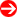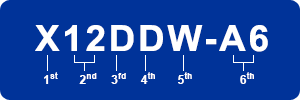#Product Naming Convention - Motherboards (Intel DP)Character Representation Options 1st + 2nd CPU Type • X11 = 11th gen. Xeon Scalable Processors         • X10 = 10th gen. Xeon (E5-2600 v4/v3)         • X9 = 9th gen. Xeon (E5-2600 v2/E5-2600) 3rd Number of CPU Supported • D = Dual 4th CPU socket / Chipset /Board Type • P = Socket P0 (LGA 3647 - X11)         • R = Socket R3 (LGA 2011 - X10)         • B = Socket B3 (LGA 1356)         • A = Workstation         • D = Datacenter Optimized         • G = GPU Optimized         • S = Storage Optimized 5th Controller Support • C = SAS3 12Gb (Broadcom)         • D = Datacenter Optimized         • F = Flexible         • FF = FatTwin Front I/O         • FR = FatTwin Rear I/O         • G = GPU Optimized         • i = SATA         • L = Cost Optimized         • Q/O = Four/Eight GPUs         • S = Storage Optimized         • T = Twin Architecture         • U = Ultra Architecture         • W = WIO Architecture         • X = Hyper-Speed or Max Expansion         • + = Extra DIMMs/Features 6th Controller Support • B = BigTwin         • BH = BigTwin with 3 UPI         • P = TwinPro         • C = SAS3 12Gb (Broadcom)         • E = BMC Enhancement (64MB)         • HGX2 = HGX2 Optimized         • i/E = SATA only         • IBQ = Infiniband QDR         • IBF = Infiniband FDR         • IBE = Infiniband EDR         • JBOD = JBOD Support         • L = Cost Optimized         • LN4 = Four LAN         • N = NVMe Support         • R = Intel Ruler NVMe         • S = SIOM Support         • T = 10GBase-T         • T4 = Four 10GBase-T         • TP = 10G SFP+         • FP = 40G SFP+         • Q/H/O = Four/Six/Eight GPUs         • q = QAT Support         • XLL = Hyper-Speed Technology         • Z = Extra Features         • + = Extra DIMMs